# near point

closest point on optical axis with an image that has „acceptable sharpness“Where CoC is the Circle of Confusion (the largest accepted Airy-disk) in Millimeter.

Alternatively, we can express the NearPoint using the magnification M :If we use for example a 1/2.5″ 5 Aptina Megapixel greyscale Sensor mit 2.2pixel pitch, we can use the pixel diagonal as CoC for crisp images, sayA 5 Mega lens with f=7.2mm focal length and F-stop F2.4, focused to an object distance of 100mm then has a far point ofund einen Nahpunkt von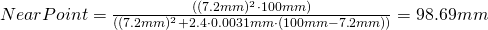and thusIf instead we use a 5 Megapixel greyscale Sony Sensor with 3.45pixel pitch, we can choose as CoC the diagonal of the pixel for crisp images, sayA 5 Mega lens with f=7.2mm focal length and F-stop F2.4, focussed to 100mm then results inmm
and a nearpoint ofthus we getIf we use a color sensor instead we can usefor crisp images. For the two sensors above we then get: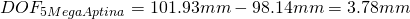To increase the DOF we can increase the Pixel Size, but we either lose resolution, or (at the same pixel count) the magnification changes)
If you change the focal length of a lens in a way, that (with the same sensor) you get the same FOV (then from a different distance) this results in the same DOF !!!
To increase the DOF we can keep the working distance and the pixel size while reducing the sensor size, but then we lose resolution (because less of the same size pixels fit on the now smaller sensor)
When a lens is focussed to the hyperfocal distance H, the far point is atand the near point is at.
The DOF isthen, thus focussing to the hyperfocal distance results in the largest possible DOF.

Yet another way to express the Near-Point is to use the Hyperfocal distance H :If the lens is then focused to some object distance OD, this OD can be expressed as fraction of H :or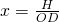Then the near point is,

the far point isand the Depth of field isWhen we set the object distance OD to the hyperfocal distance H, we get:Therefore fromwe get.
This means however, thatand therefore,For a lens with f=12mm, F#=2.8 and a CoC of 3.1um we get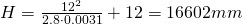Forwe getSo, withandwe get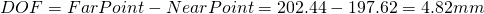Forwe getSo, withandwe getTherefore by decreasing the OD by factor 2, the DOF decreased by factor 4.

When the magnification increases by factor 2, the DOF decreases by factor 4

or, equivalent :

When the object distance decreases by factor 2, the DOF decreases by factor 4